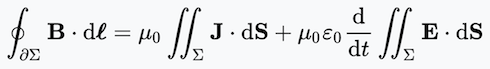### Polarized Light

#### The Mirror

I describe polarized light according to Maxwell here. I describe here some details of a polarizing filter. This was all known in Maxwell’s time and confirmed by observation.

Maxwell’s equations are differential equations and in that spirit we define a polarizing filter by posing boundary conditions in the plane z=0 thru which the the light passes. That plane is a conductor and thus a mirror. There are massless charges that can move freely in the plane and produce a current but, constrained to the plane and thus Ex = Ey = 0 and the electric field is (0, 0, Ez) at (x, y, 0). The physics might be that there are many charge carriers there that are mobile within the plane. The carriers cancel net charge and ρ=0. A solution with this boundary condition is:

For z<0

• E = (sin(z − ct) + sin(z + ct), 0, 0)
• B = (0, 0, 0).
For z>0:
• E = B = 0.
For z<0 we see another reflected light stream moving in the negative z direction and the light is entirely reflected. The magnet fields of the two streams entirely cancel; we have a standing wave. It is dark for z>0.

I must spell out the induced current in the z=0 plane!! Perhaps I need the integral equations to cope with the infinite current density on the z=0 plane.

The integral form of ∇×B = ∂E/∂t + J is:In these expressions Σ is a bounded 2D surface and ∂Σ is its boundary which is a closed loop. B is the magnetic field and E is the electric field. dS is a differential vector orthogonal to the surface Σ. μ0 and ε0 are both one here. “d” is a differential vector element of the boundary of Σ.

For Σ we choose a small square which crosses the z=0 plane. Its corners are at:
{(−0.01, 0, −0.01), (−0.01, 0, 0.01), (0.01, 0, 0.01), (0.01, 0, −0.01)}.

B∙d is 0 everywhere and thus the too left side of this equation. The vector field J is the current in the z=0 plane. J is orthogonal to dS and this term is 0 too. Just now it seems I don’t need no stinking mirror. I have made a mistake somewhere.

#### The perfect polarizing filter

Now we assume charges constrained in the z=0 plane and free to move in the x direction. The condition is now:
E(x, y, 0) = (0, Ey, Ez).